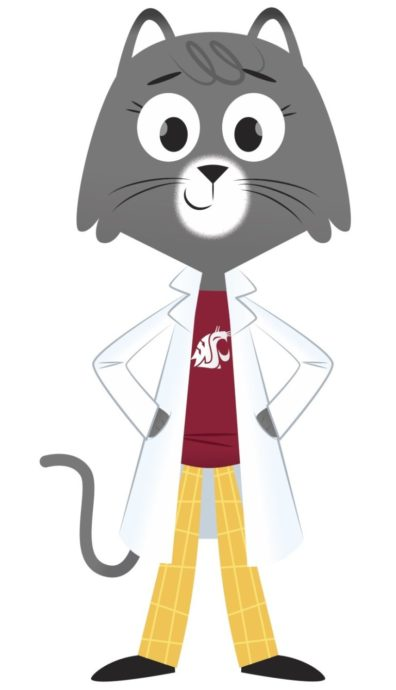Dear Keegan,

Our sun is so massive, you could fit more than one million earths inside of it. To find out how many peas would fit inside the biggest object in our solar system, I decided to ask my friend and mathematician Kimberly Vincent at Washington State University.

Vincent and her students said that to figure out how much of something can fit inside the sun, we need to know the volume of the sun. The volume is how much space something takes up.

One group of Vincent’s students worked to solve your question by estimating the volume of a pea is about 1 cubic centimeter. That’s the volume of a cube measuring 1 centimeter on each side.

We also need to know the volume of the sun. We can calculate this in kilometers. The Pacific Ocean, for example, has a volume of about 700,000,000 cubic kilometers.

The volume of the sun is about 1,410,000,000,000,000 cubic kilometers, or more than 2 million Pacific Oceans.

###Big calculations

It’s also important to know there are 100,000 centimeters in a kilometer, and 1,000,000,000,000,000 cubic centimeters in a cubic kilometer. That means we can also say the volume of the sun is 141,000,000,000,000,000,000,000 cubic centimeters.

To find out how many times a pea can fit in the sun, we divide the volume of the sun by the volume of a pea.

Here’s a quick challenge on a smaller scale. If the volume of a tennis ball is 148 cubic centimeters

and a pea is 1 cubic centimeters, how many peas could fit inside a tennis ball? Hint: You can use a calculator to type in 148 ÷ 1.

Using a similar calculation, students estimated you could fit about 141,000,000,000,000,000,000,000 peas in the sun. To me, it sounded close to a zillion peas. But it turns out a zillion isn’t a real number.

### A whole lot of zeroes

A number with two groups of three zeroes is a million. A number with three groups of three zeroes is what we call a billion. Then there’s trillion, quadrillion, quintillion, and finally sextillion (seven groups of three zeroes). We could estimate 141 sextillion peas could fit in the sun.

After sextillion comes septillion, octillion, nonillion, decillion, and it goes on and on. These big numbers are useful when we want to make estimates or measure the size of the universe, the space between stars, or finding out how much water fills up a lake or ocean.

Vincent’s students also reminded me that sometimes we can approach an answer to a question in different ways. Maybe you have a different sized pea or you want to use inches or feet in your calculations. A few of the students were also thinking outside the box with your question. One of the students said that no peas would fit in the sun because the sun is a nuclear reactor and would make the peas disintegrate. Another student said it depends on how you hold the pea—if you hold it close to your eye, it might just take one to fill up, or cover, the whole sun.

Sincerely,
Dr. Universe